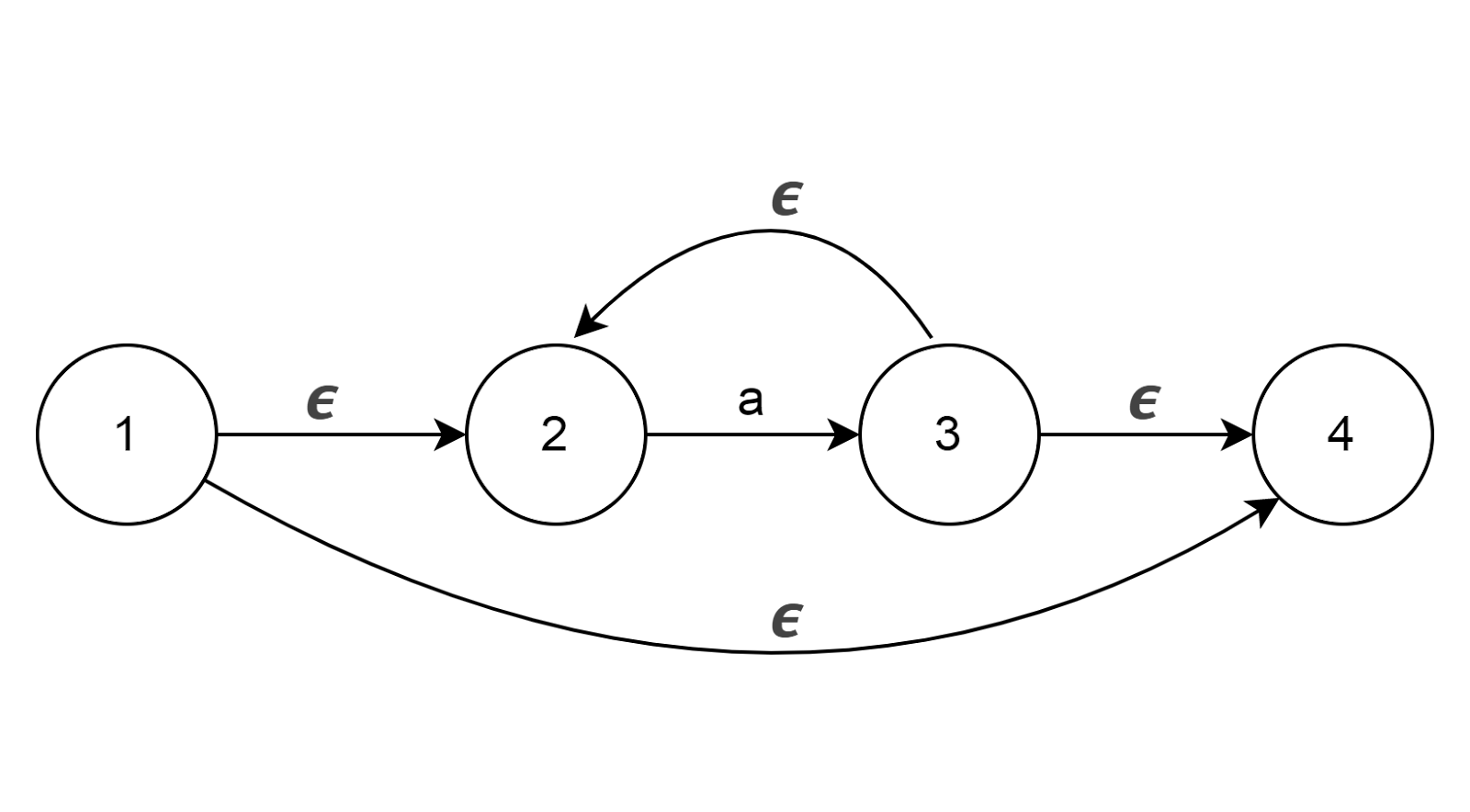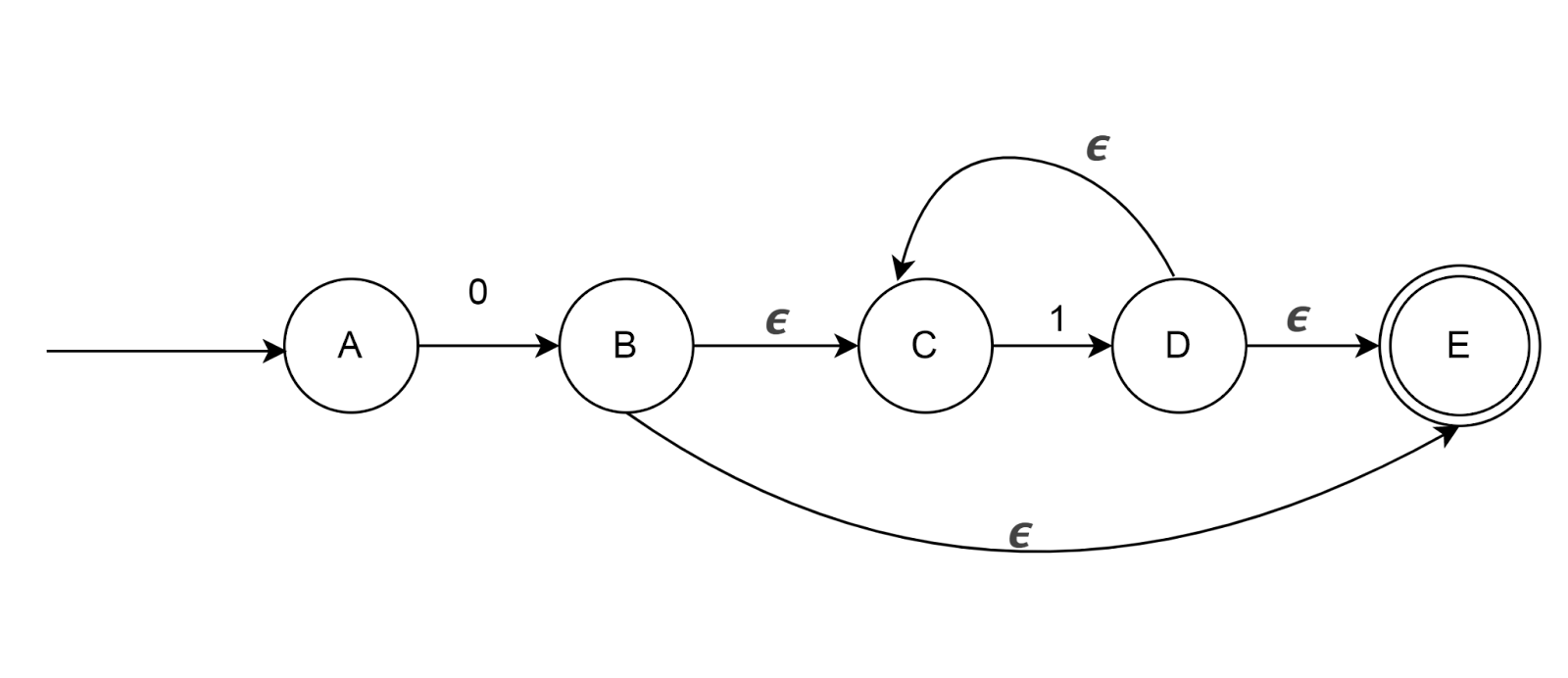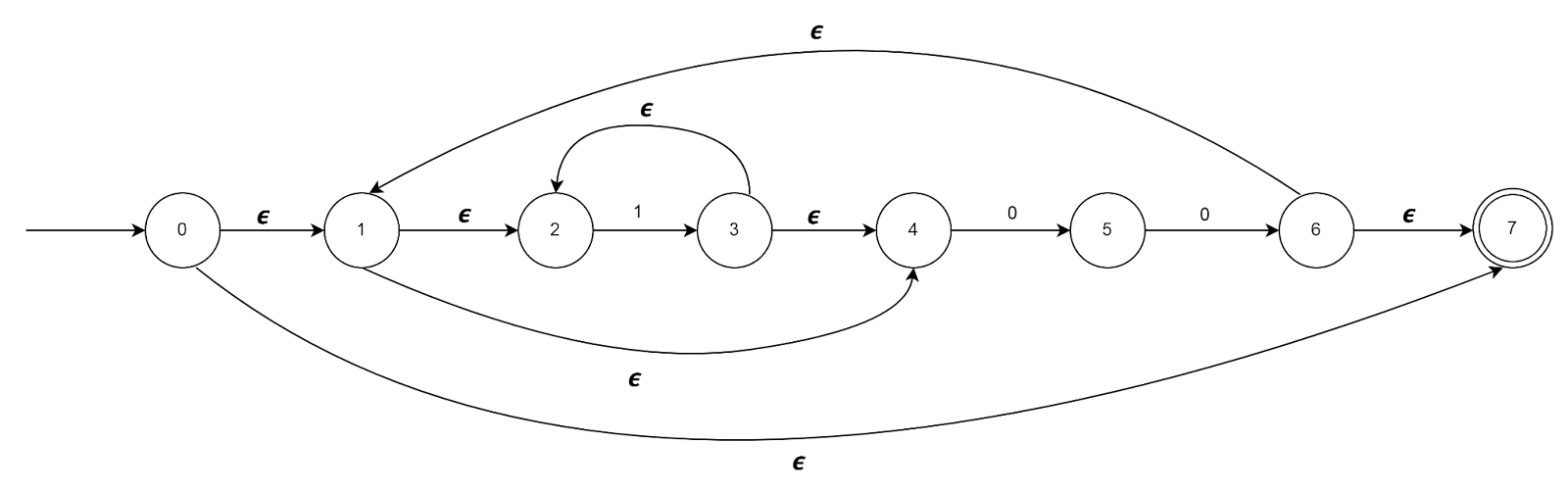• Construct NFA for the following Regular Expressions
1. ba*b

2. (a|b)c

3. a(bc)*

4. (a/b)*(abb|a+b)

5. 1*(001+)*

6. (0+1)*01(0+1)*

• Construct DFA for the following Expressions
1. (a|b|c)*bca(a|b|c)*
2. L={ (ab)n |n≥ 0}
3. a*ab
4. 101(0|1)*
5. Set of all strings with three consecutive 0’s
6. (0+1)*0011

Thompson Construction Method

1. Thompson construction method is used in constructing the 𝟄-Non Deterministic Finite Automata (𝟄-NFA) from regular expression

3. This algorithm recursively splits the regular expression into subexpressions and the NFA is constructed from it using certain rules.

Rules defining the NFA

1. Concatenation (ab)1. Union (a+b)1. Closure (a*)Examples:

1. Construct NFA for 01* using Thompson Construction Method1. Construct NFA for (1*00)* using Thompson Construction Method